# Algebraic tangles

(diff) ← Older revision | Latest revision (diff) | Newer revision → (diff)

A family of tangles (cf. Tangle) defined recursively for anyas follows:

i)-algebraic tangles is the smallest family of-tangles satisfying

1) any-tangle withorcrossing is-algebraic;

2) ifandare-algebraic tangles, then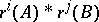is-algebraic for any integers,, wheredenotes the rotation of a tangle by the angle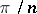anddenotes (horizontal) composition of tangles.

ii) If in condition 2) above,is restricted to tangles with no more thancrossings, one obtains the family of-algebraic tangles.

iii) If an-tangle,, is obtained from an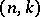-algebraic tangle (respectively, an-algebraic tangle) by partially closing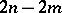of its endpoints without a crossing, thenis called an-algebraic-tangle, respectively an-algebraic-tangle. For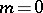one obtains an-algebraic link, respectively an-algebraic link.-algebraic tangles were introduced by J.H. Conway (they are often called algebraic tangles in the sense of Conway or arborescent tangles). The-fold branched covering ofwith a-algebraic link as a branched set is a Waldhausen graph manifold. Thus, not every link is-algebraic. It is an open problem (as of 2001) to find, for a given, a link which is not-algebraic. The smallestfor which a link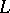is-algebraic is called the algebraic index of the link (it is bounded from above by the braid and bridge indices of the link). For example, the algebraic index of the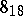knot is equal to.

How to Cite This Entry:
Algebraic tangles. Encyclopedia of Mathematics. URL: http://encyclopediaofmath.org/index.php?title=Algebraic_tangles&oldid=14501
This article was adapted from an original article by Jozef Przytycki (originator), which appeared in Encyclopedia of Mathematics - ISBN 1402006098. See original article